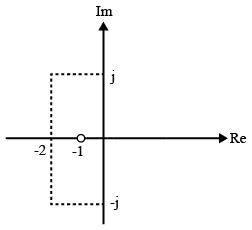# ISRO Scientist or Engineer Electronics 2009

Instructions

For the following questions answer them individually

Question 1

# If a counter having 10 FFsis initially at 0, what countwill it hold after 2060 pulses?Question 2

# Amemory system of size 16 K bytes is required to be designed using memory chips which have 12 address lines and 4 datalines each. Then the numberofsuch chips required to design the memory system isQuestion 3

# Consider the following program for 8085XRAALXI B, 0007HLOOP: DCXBJNZ LOOPThe loop will be executedQuestion 4

# Acertain JK FF has $$t_{pd}$$=12 ns. The largest MOD counter that can be constructed from such FFs and still operate up to 10 MHz isQuestion 5

# Convolution of x(t+5) with impulse function $$\delta$$ (t-7) is equal toQuestion 6

# The purpose of a Cassegrain feed in a parabolic reflector antenna is toQuestion 7

# A transfer function G(s) has pole-zero plot as Im "shown in the figure. Given that the steady state function gain is 2,, the transfer function A G(s) will be given byQuestion 8

# The impedance of a series RLC circuit isQuestion 9

# The advantage of $$\frac{\pi}{4}$$ QPSK compared to conventional QPSKis ?Question 10

# A movingcoil iron ammeter may be compensated for frequency errors byOR